Скачать презентацию Financial Sector Money Market AP Economics Mr Bordelon

c2991996b0a2c49d054fdd636a7074f6.ppt

• Количество слайдов: 17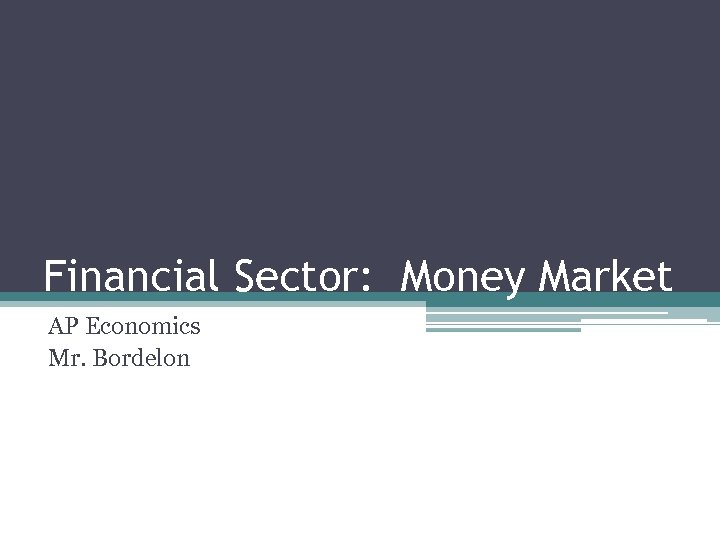Financial Sector: Money Market AP Economics Mr. Bordelon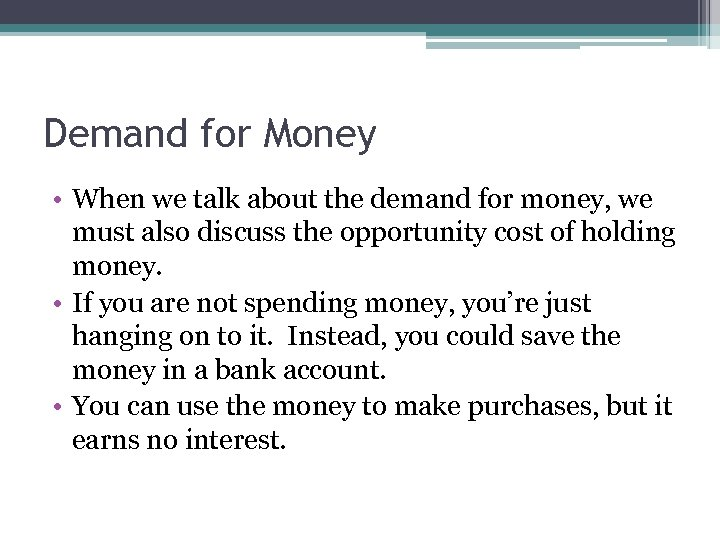Demand for Money • When we talk about the demand for money, we must also discuss the opportunity cost of holding money. • If you are not spending money, you’re just hanging on to it. Instead, you could save the money in a bank account. • You can use the money to make purchases, but it earns no interest.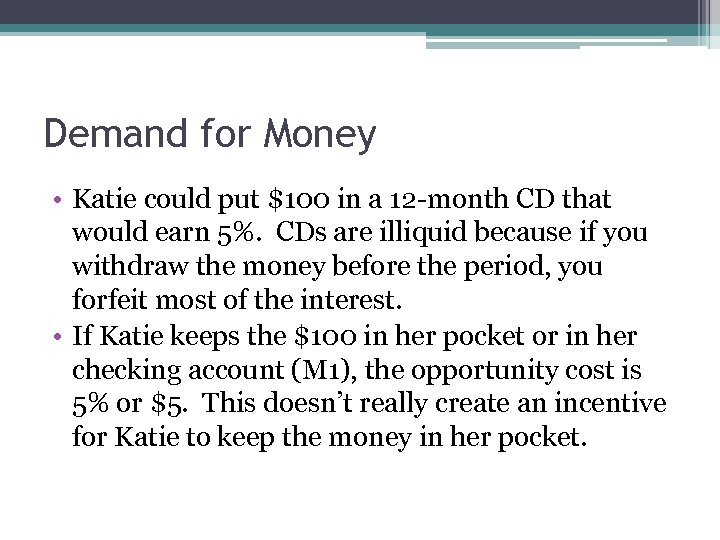Demand for Money • Katie could put \$100 in a 12 -month CD that would earn 5%. CDs are illiquid because if you withdraw the money before the period, you forfeit most of the interest. • If Katie keeps the \$100 in her pocket or in her checking account (M 1), the opportunity cost is 5% or \$5. This doesn’t really create an incentive for Katie to keep the money in her pocket.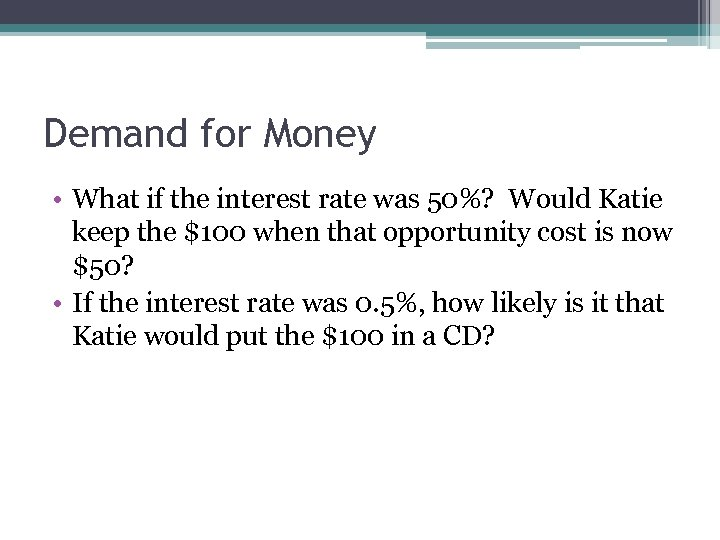Demand for Money • What if the interest rate was 50%? Would Katie keep the \$100 when that opportunity cost is now \$50? • If the interest rate was 0. 5%, how likely is it that Katie would put the \$100 in a CD?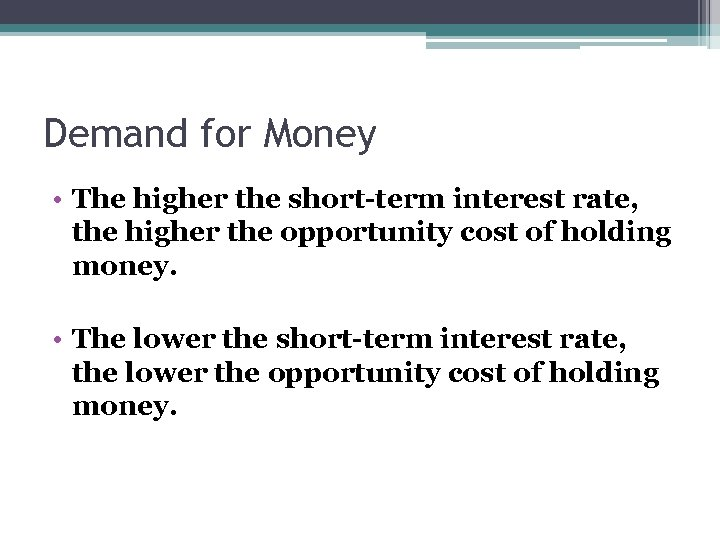Demand for Money • The higher the short-term interest rate, the higher the opportunity cost of holding money. • The lower the short-term interest rate, the lower the opportunity cost of holding money.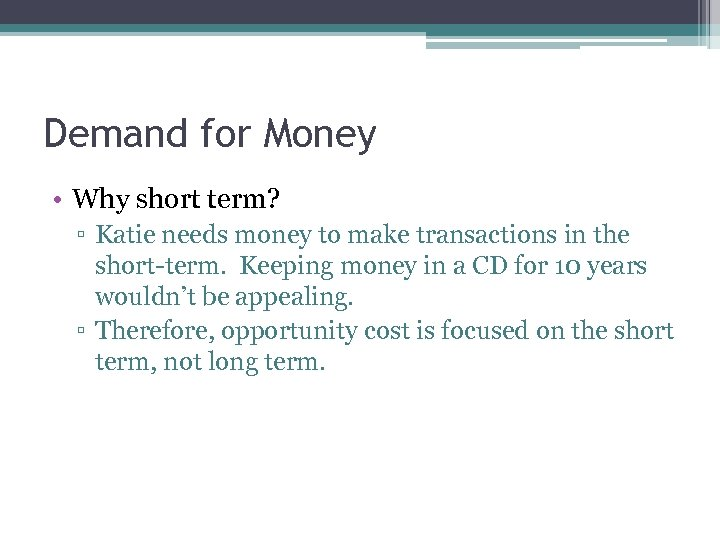Demand for Money • Why short term? ▫ Katie needs money to make transactions in the short-term. Keeping money in a CD for 10 years wouldn’t be appealing. ▫ Therefore, opportunity cost is focused on the short term, not long term.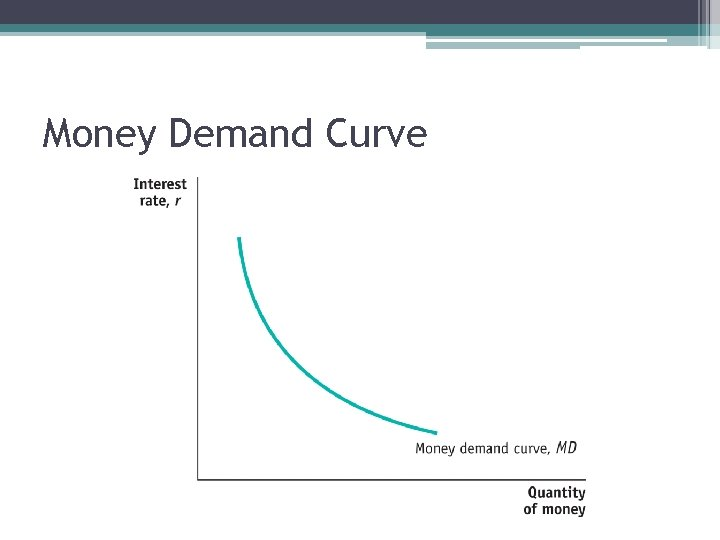Money Demand Curve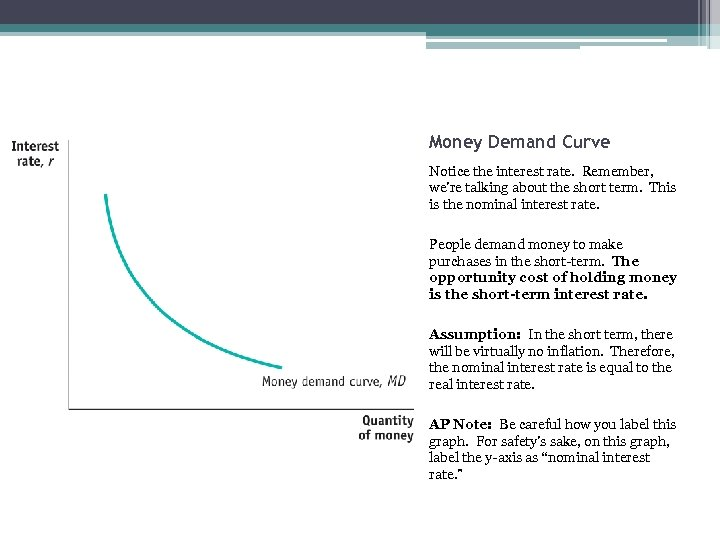Money Demand Curve Notice the interest rate. Remember, we’re talking about the short term. This is the nominal interest rate. People demand money to make purchases in the short-term. The opportunity cost of holding money is the short-term interest rate. Assumption: In the short term, there will be virtually no inflation. Therefore, the nominal interest rate is equal to the real interest rate. AP Note: Be careful how you label this graph. For safety’s sake, on this graph, label the y-axis as “nominal interest rate. ”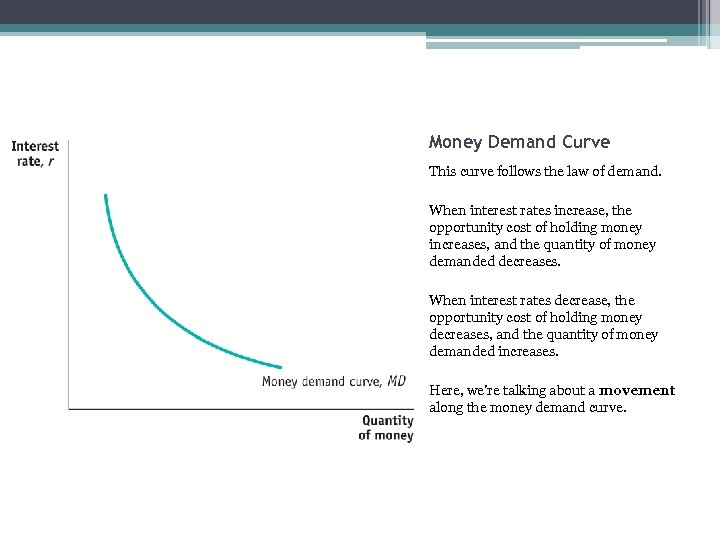Money Demand Curve This curve follows the law of demand. When interest rates increase, the opportunity cost of holding money increases, and the quantity of money demanded decreases. When interest rates decrease, the opportunity cost of holding money decreases, and the quantity of money demanded increases. Here, we’re talking about a movement along the money demand curve.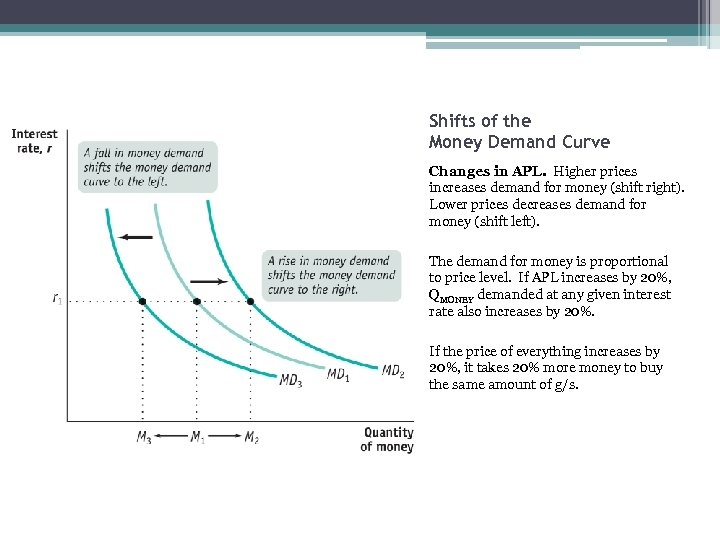Shifts of the Money Demand Curve Changes in APL. Higher prices increases demand for money (shift right). Lower prices decreases demand for money (shift left). The demand for money is proportional to price level. If APL increases by 20%, QMONEY demanded at any given interest rate also increases by 20%. If the price of everything increases by 20%, it takes 20% more money to buy the same amount of g/s.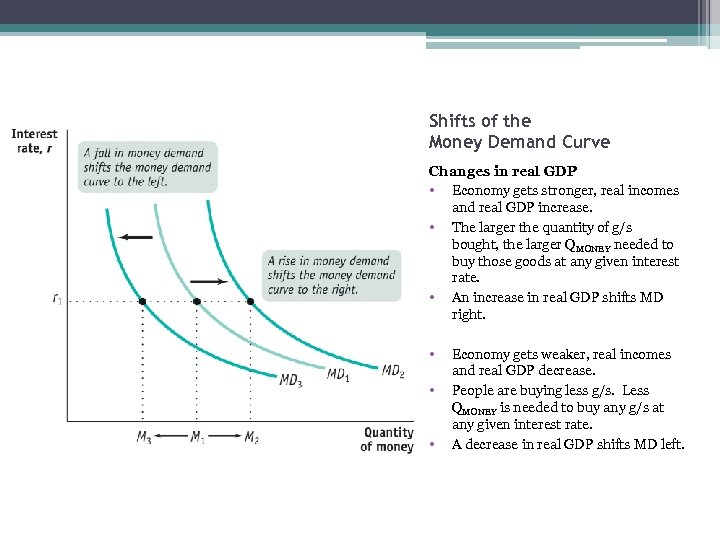Shifts of the Money Demand Curve Changes in real GDP • Economy gets stronger, real incomes and real GDP increase. • The larger the quantity of g/s bought, the larger QMONEY needed to buy those goods at any given interest rate. • An increase in real GDP shifts MD right. • • • Economy gets weaker, real incomes and real GDP decrease. People are buying less g/s. Less QMONEY is needed to buy any g/s at any given interest rate. A decrease in real GDP shifts MD left.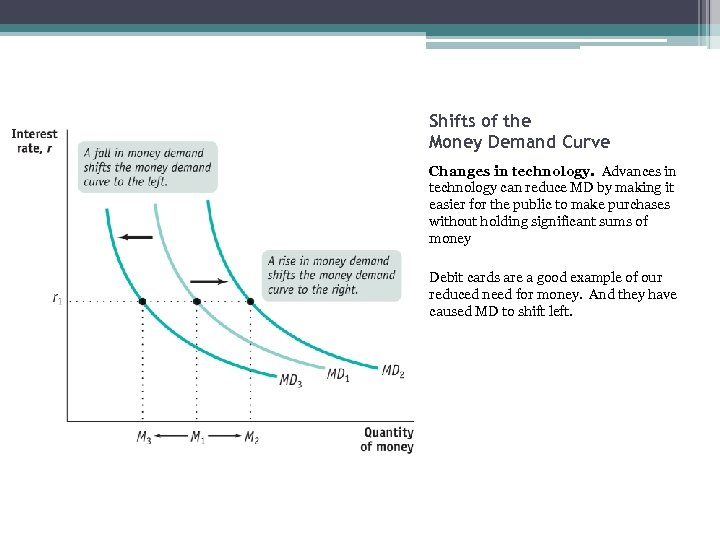Shifts of the Money Demand Curve Changes in technology. Advances in technology can reduce MD by making it easier for the public to make purchases without holding significant sums of money Debit cards are a good example of our reduced need for money. And they have caused MD to shift left.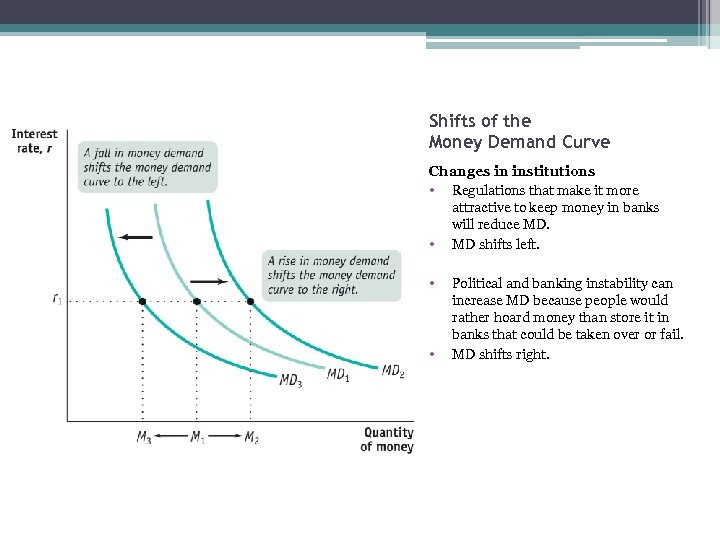Shifts of the Money Demand Curve Changes in institutions • Regulations that make it more attractive to keep money in banks will reduce MD. • MD shifts left. • • Political and banking instability can increase MD because people would rather hoard money than store it in banks that could be taken over or fail. MD shifts right.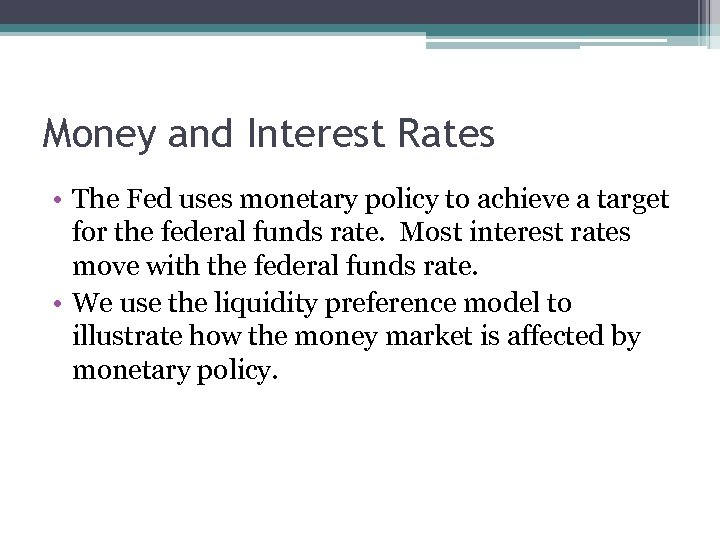Money and Interest Rates • The Fed uses monetary policy to achieve a target for the federal funds rate. Most interest rates move with the federal funds rate. • We use the liquidity preference model to illustrate how the money market is affected by monetary policy.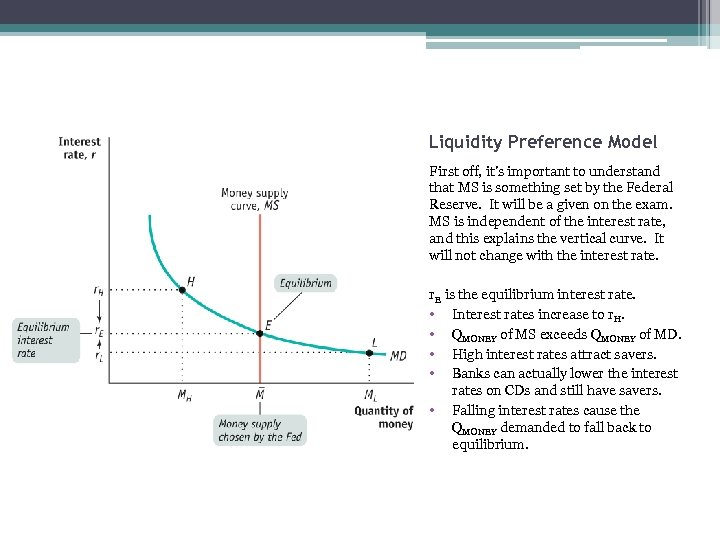Liquidity Preference Model First off, it’s important to understand that MS is something set by the Federal Reserve. It will be a given on the exam. MS is independent of the interest rate, and this explains the vertical curve. It will not change with the interest rate. r. E is the equilibrium interest rate. • Interest rates increase to r. H. • QMONEY of MS exceeds QMONEY of MD. • High interest rates attract savers. • Banks can actually lower the interest rates on CDs and still have savers. • Falling interest rates cause the QMONEY demanded to fall back to equilibrium.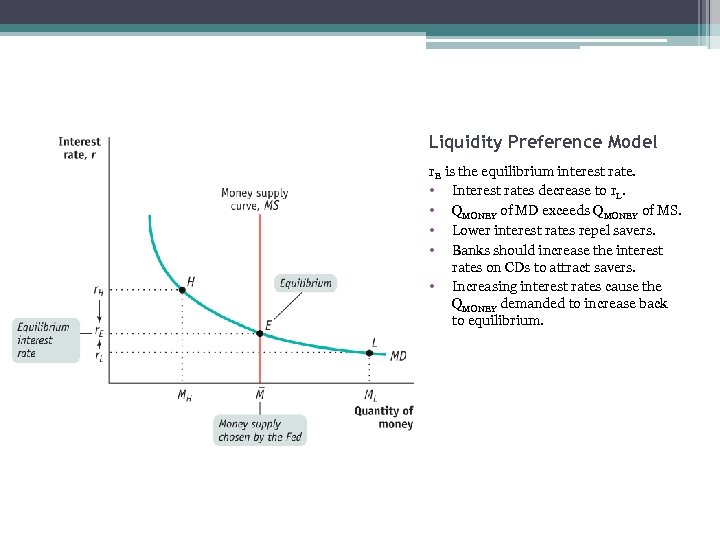Liquidity Preference Model r. E is the equilibrium interest rate. • Interest rates decrease to r. L. • QMONEY of MD exceeds QMONEY of MS. • Lower interest rates repel savers. • Banks should increase the interest rates on CDs to attract savers. • Increasing interest rates cause the QMONEY demanded to increase back to equilibrium.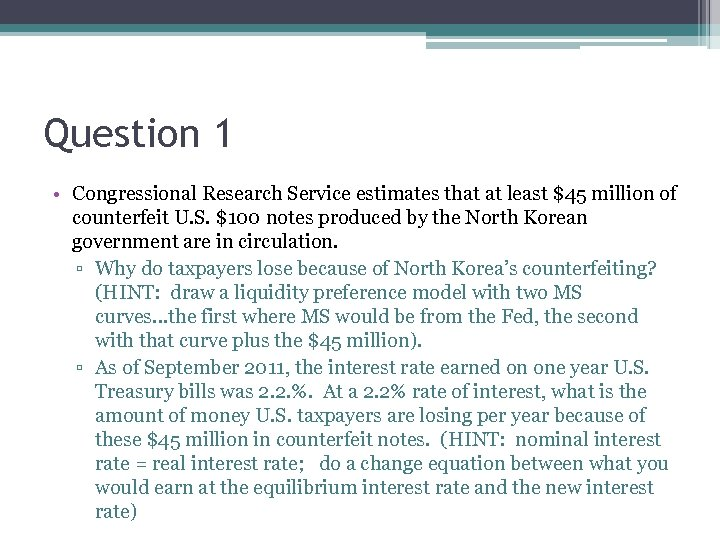Question 1 • Congressional Research Service estimates that at least \$45 million of counterfeit U. S. \$100 notes produced by the North Korean government are in circulation. ▫ Why do taxpayers lose because of North Korea’s counterfeiting? (HINT: draw a liquidity preference model with two MS curves…the first where MS would be from the Fed, the second with that curve plus the \$45 million). ▫ As of September 2011, the interest rate earned on one year U. S. Treasury bills was 2. 2. %. At a 2. 2% rate of interest, what is the amount of money U. S. taxpayers are losing per year because of these \$45 million in counterfeit notes. (HINT: nominal interest rate = real interest rate; do a change equation between what you would earn at the equilibrium interest rate and the new interest rate)